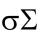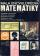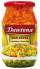Infirmary

Two thirds of children from the infirmary went on a trip seventh went to bathe and 40 children remained in the gym. How many children were treated in the infirmary?

Result

x =  210

Solution:

2/3x + 1/7 x + 40 = x

4x = 840

x = 210

Calculated by our simple equation calculator.

Leave us a comment of this math problem and its solution (i.e. if it is still somewhat unclear...):Be the first to comment!To solve this verbal math problem are needed these knowledge from mathematics:

Do you have a linear equation or system of equations and looking for its solution? Or do you have quadratic equation?

Next similar math problems:

1. Dropped sheetsThree consecutive sheets dropped from the book. The sum of the numbers on the pages of the dropped sheets is 273. What number has the last page of the dropped sheets?
2. Three numbersCreate from digits 1-9 three-digit numbers with their sum the smallest. What value is the sum of these numbers? (Use each digit only once)
3. NormThree workers planted 3555 seedlings of tomatoes in one dey. First worked at the standard norm, the second planted 120 seedlings more and the third 135 seedlings more than the first worker. How many seedlings were standard norm?
4. BookTo number pages of thick book was used 4201 digits. How many pages has this book?
5. Simple equation 9Solve the following equation: -8y+5=-9y+9
6. Forest nurseryIn the forest nursery after winter, they found that 1/10 stems died out of them. For them, they land 193 new spruces. How many spruces are in the forest nursery?
7. Simple equation 8Solve the following equation: 36=-(1+7x)-6(-7-x)
8. Simple equationSolve the following simple equation: 2. (4x + 3) = 2-5. (1-x)
9. Tickets 3A total of 645 tickets were sold for the school play. They were either adult tickets or student tickets. There were 55 fewer student tickets sold than adult tickets. How many adult tickets were sold?
10. Unknown number 11That number increased by three equals three times itself?
11. Find xSolve: if 2(x-1)=14, then x= (solve an equation with one unknown)
12. Simple equationSolve for x: 3(x + 2) = x - 18
13. Equation 29Solve next equation: 2 ( 2x + 3 ) = 8 ( 1 - x) -5 ( x -2 )
14. Negative in equation2x + 3 + 7x = – 24, what is the value of x?
15. Unknown numberDetermine the unknown number that is equal to a quarter of the fifth of number, which is by 152 more than unknown number.
16. Last pageTwo consecutive sheets dropped out of the book. The sum of the numbers on the sides of the dropped sheets is 154. What is the number of the last page of the dropped sheets?
17. The storeThe store received the same number of cans of peas and corn. The first day sold 10 cans of peas and 166 cans ofcorn so that left 5 times more peas than corn cans. How many cans of each kind were in the store?